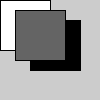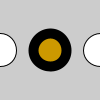# push_matrix()#

Pushes the current transformation matrix onto the matrix stack.

## Examples#def setup():
py5.fill(255)
py5.rect(0, 0, 50, 50)  # white rectangle

py5.push_matrix()
py5.translate(30, 20)
py5.fill(0)
py5.rect(0, 0, 50, 50)  # black rectangle
py5.pop_matrix()

py5.fill(100)
py5.rect(15, 10, 50, 50)  # gray rectangledef setup():
py5.ellipse(0, 50, 33, 33)  # left circle

py5.stroke_weight(10)
py5.fill(204, 153, 0)

with py5.push():
py5.translate(50, 0)
py5.ellipse(0, 50, 33, 33)  # middle circle

py5.stroke_weight(1)
py5.fill(255)
py5.ellipse(100, 50, 33, 33)  # right circle


## Description#

Pushes the current transformation matrix onto the matrix stack. Understanding push_matrix() and pop_matrix() requires understanding the concept of a matrix stack. The push_matrix() function saves the current coordinate system to the stack and pop_matrix() restores the prior coordinate system. push_matrix() and pop_matrix() are used in conjuction with the other transformation functions and may be embedded to control the scope of the transformations.

This method can be used as a context manager to ensure that pop_matrix() always gets called, as shown in the last example.

Underlying Processing method: pushMatrix

## Signatures#

push_matrix() -> None


Updated on March 06, 2023 02:49:26am UTC